### Practical Geometry-Solutions 14.5

CBSE Class –VI Mathematics
NCERT Solutions
Chaper 14 Practical Geometry (Ex. 14.5)

Question 1. Draw $\overline{\text{AB}}$ of length 7.3 cm and find its axis of symmetry.
Answer: Axis of symmetry of line segment $\overline{\text{AB}}$ will be the perpendicular bisector of $\overline{\text{AB}}.$ So, draw the perpendicular bisector of AB.
Steps of construction: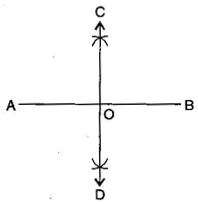(i) Draw a line segment $\overline{\text{AB}}$ = 7.3 cm
(ii) Taking A and B as centres and radius more than half of AB, draw two arcs which intersect each other at C and D.
(iii) Join CD. Then CD is the axis of symmetry of the line segment AB.

Question 2. Draw a line segment of length 9.5 cm and construct its perpendicular bisector.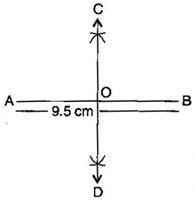(i) Draw a line segment $\overline{\text{AB}}$ = 9.5 cm
(ii) Taking A and B as centres and radius more than half of AB, draw two arcs which intersect each other at C and D.
(iii) Join CD. Then CD is the perpendicular bisector of $\overline{\text{AB}}$.
Question 3. Draw the perpendicular bisector of $\overline{\text{XY}}$ whose length is 10.3 cm.
(a) Take any point P on the bisector drawn. Examine whether PX = PY.
(b) If M is the mid-point of $\overline{\text{XY}}$, what can you say about the lengths MX and XY?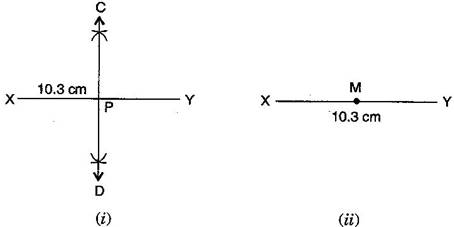(i) Draw a line segment $\overline{\text{XY}}$ = 10.3 cm
(ii) Taking X and Y as centres and radius more than half of XY, draw two arcs which intersect each other at C and D.
(iii) Join CD. Then CD is the required perpendicular bisector of $\overline{\text{XY}}$.
Now:
(a) Take any point P on the bisector drawn. With the help of divider we can check that $\overline{\text{PX}}=\overline{\text{PY}}$.
(b) If M is the mid-point of $\overline{\text{XY}},$ then $\overline{\text{MX}}=\frac{1}{2}\overline{\text{XY}}$.
Question 4. Draw a line segment of length 12.8 cm. Using compasses, divide it into four equal parts. Verify by actual measurement.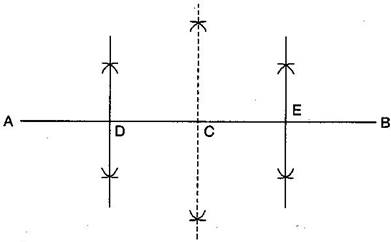(i) Draw a line segment AB = 12.8 cm
(ii) Draw the perpendicular bisector of $\overline{\text{AB}}$ which cuts it at C. Thus, C is the mid-point of $\overline{\text{AB}}.$
(iii) Draw the perpendicular bisector of $\overline{\text{AC}}$ which cuts it at D. Thus D is the mid-point of $\overline{\text{AC}}$ .
(iv) Again, draw the perpendicular bisector of $\overline{\text{CB}}$ which cuts it at E. Thus, E is the mid-point of $\overline{\text{CB}}$.
(v) Now, point C, D and E divide the line segment $\overline{\text{AB}}$ in the four equal parts.
(vi) By actual measurement, we find that
$\overline{\text{AD}}=\overline{\text{DC}}=\overline{\text{CE}}=\overline{\text{EB}}=3.2$ cm
Question 5.With $\overline{\text{PQ}}$ of length 6.1 cm as diameter, draw a circle.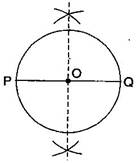(i) Draw a line segment $\overline{\text{PQ}}$ = 6.1 cm.
(ii) Draw the perpendicular bisector of PQ which cuts, it at O. Thus O is the mid-point of $\overline{\text{PQ}}$.
Taking O as centre and OP or OQ as radius draw a circle where diameter is the line segment $\overline{\text{PQ}}$.
Question 6.Draw a circle with centre C and radius 3.4 cm. Draw any chord $\overline{\text{AB}}.$ Construct the perpendicular bisector $\overline{\text{AB}}$ and examine if it passes through C.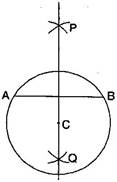(i) Draw a circle with centre C and radius 3.4 cm.
(ii) Draw any chord $\overline{\text{AB}}.$
(iii) Taking A and B as centers and radius more than half of $\overline{\text{AB}},$ draw two arcs which cut each other at P and Q.
(iv) Join PQ. Then PQ is the perpendicular bisector of $\overline{\text{AB}}.$
This perpendicular bisector of $\overline{\text{AB}}$ passes through the centre C of the circle.
Question 7.Repeat Question 6, if $\overline{\text{AB}}$ happens to be a diameter.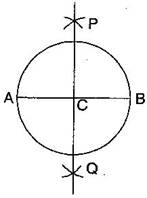(i) Draw a circle with centre C and radius 3.4 cm.
(ii) Draw its diameter $\overline{\text{AB}}$.
(iii) Taking A and B as centers and radius more than half of it, draw two arcs which intersect each other at P and Q.
(iv) Join PQ. Then PQ is the perpendicular bisector of $\overline{\text{AB}}$.
We observe that this perpendicular bisector of $\overline{\text{AB}}$ passes through the centre C of the circle.
Question 8.Draw a circle of radius 4 cm. Draw any two of its chords. Construct the perpendicular bisectors of these chords. Where do they meet?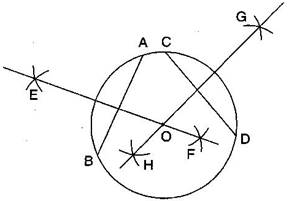(i) Draw the circle with O and radius 4 cm.
(ii) Draw any two chords $\overline{\text{AB}}$ and $\overline{\text{CD}}$ in this circle.
(iii) Taking A and B as centers and radius more than half AB, draw two arcs which intersect each other at E and F.
(iv) Join EF. Thus EF is the perpendicular bisector of chord  $\overline{\text{AB}}$ .
(v) Similarly draw GH the perpendicular bisector of chord $\overline{\text{CD}}$.
These two perpendicular bisectors meet at O, the centre of the circle.
Question 9.Draw any angle with vertex O. Take a point A on one of its arms and B on another such that OA = OB. Draw the perpendicular bisectors of $\overline{\text{OA}}$ and $\overline{\text{OB}}.$ Let them meet at P. Is PA = PB?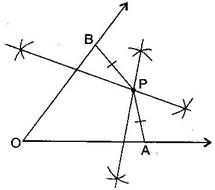(iii) Draw perpendicular bisector of $\overline{\text{OA}}$ and $\overline{\text{OB}}.$